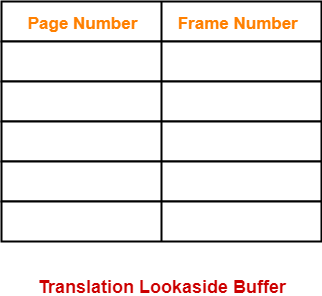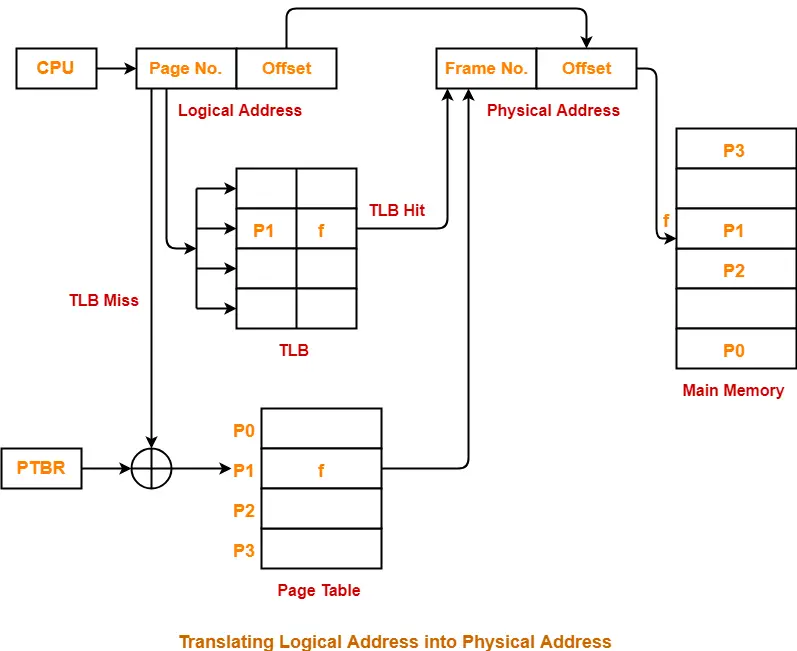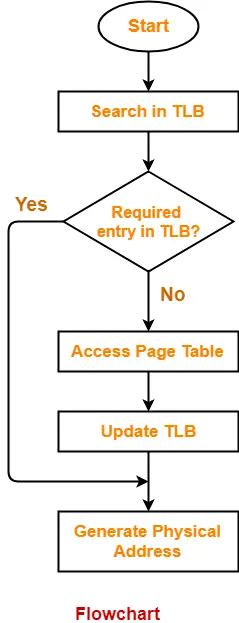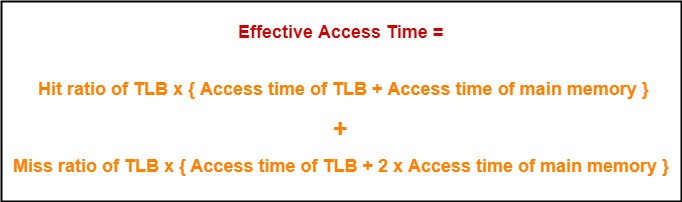# Translation Lookaside Buffer | TLB | Paging

## Paging in OS-

Before you go through this article, make sure that you have gone through the previous article on Paging in OS.

We have discussed-

• Paging in OS is a non-contiguous memory allocation technique.
• Page Table is a data structure that maps page number to the frame number.

One major disadvantage of paging is-

• It increases the effective access time due to increased number of memory accesses.
• One memory access is required to get the frame number from the page table.
• Another memory access is required to get the word from the page.

## Translation Lookaside Buffer-

• Translation Lookaside Buffer (TLB) is a solution that tries to reduce the effective access time.
• Being a hardware, the access time of TLB is very less as compared to the main memory.

## Structure-

Translation Lookaside Buffer (TLB) consists of two columns-

1. Page Number
2. Frame NumberIn a paging scheme using TLB,

The logical address generated by the CPU is translated into the physical address using following steps-

## Step-01:

CPU generates a logical address consisting of two parts-

1. Page Number
2. Page Offset

## Step-02:

• TLB is checked to see if it contains an entry for the referenced page number.
• The referenced page number is compared with the TLB entries all at once.

Now, two cases are possible-

### Case-01: If there is a TLB hit-

• If TLB contains an entry for the referenced page number, a TLB hit occurs.
• In this case, TLB entry is used to get the corresponding frame number for the referenced page number.

### Case-02: If there is a TLB miss-

• If TLB does not contain an entry for the referenced page number, a TLB miss occurs.
• In this case, page table is used to get the corresponding frame number for the referenced page number.
• Then, TLB is updated with the page number and frame number for future references.

## Step-03:

• After the frame number is obtained, it is combined with the page offset to generate the physical address.
• Then, physical address is used to read the required word from the main memory.

## NOTE-

In the above discussion, we have assumed that no Page Fault occurs.

## Diagram-

The following diagram illustrates the above steps of translating logical address into physical address-## Flowchart-

The following flowchart illustrates the above steps of translating logical address into physical address-## Important Points-

### Point-01:

• Unlike page table, there exists only one TLB in the system.
• So, whenever context switching occurs, the entire content of TLB is flushed and deleted.
• TLB is then again updated with the currently running process.

### Point-02:

When a new process gets scheduled-

• Initially, TLB is empty. So, TLB misses are frequent.
• With every access from the page table, TLB is updated.
• After some time, TLB hits increases and TLB misses reduces.

### Point-03:

• The time taken to update TLB after getting the frame number from the page table is negligible.
• Also, TLB is updated in parallel while fetching the word from the main memory.

The advantages of using TLB are-

• TLB reduces the effective access time.
• Only one memory access is required when TLB hit occurs.

A major disadvantage of using TLB is-

• After some time of running the process, when TLB hits increases and process starts to run smoothly, a context switching occurs.
• The entire content of the TLB is flushed.
• Then, TLB is again updated with the currently running process.

This happens again and again.

• TLB can hold the data of only one process at a time.
• When context switches occur frequently, the performance of TLB degrades due to low hit ratio.
• As it is a special hardware, it involves additional cost.

## Effective Access Time-

In a single level paging using TLB, the effective access time is given as-This formula is valid only when there is single level paging and there are no page faults.

## Problem-01:

A paging scheme uses a Translation Lookaside buffer (TLB). A TLB access takes 10 ns and a main memory access takes 50 ns. What is the effective access time (in ns) if the TLB hit ratio is 90% and there is no page fault?

1. 54
2. 60
3. 65
4. 75

## Solution-

Given-

• TLB access time = 10 ns
• Main memory access time = 50 ns
• TLB Hit ratio = 90% = 0.9

### Calculating TLB Miss Ratio-

TLB Miss ratio

= 1 – TLB Hit ratio

= 1 – 0.9

= 0.1

### Calculating Effective Access Time-

Substituting values in the above formula, we get-

Effective Access Time

= 0.9 x { 10 ns + 50 ns } + 0.1 x { 10 ns + 2 x 50 ns }

= 0.9 x 60 ns + 0.1 x 110 ns

= 54 ns + 11 ns

= 65 ns

Thus, Option (C) is correct.

## Problem-02:

A paging scheme uses a Translation Lookaside buffer (TLB). The effective memory access takes 160 ns and a main memory access takes 100 ns. What is the TLB access time (in ns) if the TLB hit ratio is 60% and there is no page fault?

1. 54
2. 60
3. 20
4. 75

## Solution-

Given-

• Effective access time = 160 ns
• Main memory access time = 100 ns
• TLB Hit ratio = 60% = 0.6

### Calculating TLB Miss Ratio-

TLB Miss ratio

= 1 – TLB Hit ratio

= 1 – 0.6

= 0.4

### Calculating TLB Access Time-

Let TLB access time = T ns.

Substituting values in the above formula, we get-

160 ns = 0.6 x { T + 100 ns } + 0.4 x { T + 2 x 100 ns }

160 = 0.6 x T + 60 + 0.4 x T + 80

160 = T + 140

T = 160 – 140

T = 20

Thus, Option (C) is correct.

To gain better understanding about Translation Lookaside Buffer (TLB),

Watch this Video Lecture

Next Article- Multilevel Paging

Get more notes and other study material of Operating System.

Watch video lectures by visiting our YouTube channel LearnVidFun.

SummaryArticle Name
Translation Lookaside Buffer | TLB | Paging
Description
In paging, Translation Lookaside Buffer or TLB is a solution that tries to reduce the effective access time. Paging in OS using TLB requires only one memory reference if TLB hit occurs.
Author
Publisher Name
Gate Vidyalay
Publisher Logo